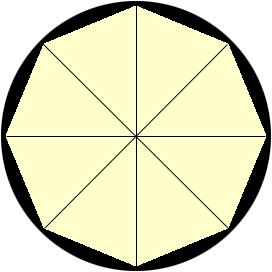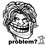# Combinatorics-RMO 2015Suppose there are 36 equally spaced points on the circumference of a circle.In how many ways you can choose 3 any two points such that no 2 of them are adjacent or diametrically opposite?Note by Souryajit Roy
5 years, 6 months ago

This discussion board is a place to discuss our Daily Challenges and the math and science related to those challenges. Explanations are more than just a solution — they should explain the steps and thinking strategies that you used to obtain the solution. Comments should further the discussion of math and science.

When posting on Brilliant:

• Use the emojis to react to an explanation, whether you're congratulating a job well done , or just really confused .
• Ask specific questions about the challenge or the steps in somebody's explanation. Well-posed questions can add a lot to the discussion, but posting "I don't understand!" doesn't help anyone.
• Try to contribute something new to the discussion, whether it is an extension, generalization or other idea related to the challenge.
• Stay on topic — we're all here to learn more about math and science, not to hear about your favorite get-rich-quick scheme or current world events.

MarkdownAppears as
*italics* or _italics_ italics
**bold** or __bold__ bold
- bulleted- list
• bulleted
• list
1. numbered2. list
1. numbered
2. list
Note: you must add a full line of space before and after lists for them to show up correctly
paragraph 1paragraph 2

paragraph 1

paragraph 2

[example link](https://brilliant.org)example link
> This is a quote
This is a quote
    # I indented these lines
# 4 spaces, and now they show
# up as a code block.

print "hello world"
# I indented these lines
# 4 spaces, and now they show
# up as a code block.

print "hello world"
MathAppears as
Remember to wrap math in $$ ... $$ or $ ... $ to ensure proper formatting.
2 \times 3 $2 \times 3$
2^{34} $2^{34}$
a_{i-1} $a_{i-1}$
\frac{2}{3} $\frac{2}{3}$
\sqrt{2} $\sqrt{2}$
\sum_{i=1}^3 $\sum_{i=1}^3$
\sin \theta $\sin \theta$
\boxed{123} $\boxed{123}$

## Comments

Sort by:

Top Newest

We shall use PIE.

Step 1: Number of ways of selecting $3$ points from $36$ points is $\binom{36}{3} = 7140$.

Step 2: Number of ways of selecting $3$ adjacent points is $36$.

Step 3: Number of ways of selecting $2$ adjacent and one not adjacent with them is $36\times 32 = 1152$. (Since there are $32$ ways to select the non-adjacent point.)

Step 4: Number of ways of selecting two diametrically opposite points are $18$ and number of ways of selecting third one not adjacent to both of them are $30$ in each case. So total number of ways in this step are $18 \times 30 = 540$.

Step 5: Number of ways (what we required) = Total $-$ Number of ways of selecting $3$ adjacent points $-$ Number of ways of selecting $2$ adjacent and one not adjacent with them - Number of ways of selecting two diametrically opposite points and selecting third one not adjacent to both of them $= 7140-36-1152-540=5412$.

- 5 years, 6 months ago

Log in to reply

I think there were 32 points instead of 36.

- 5 years, 6 months ago

Log in to reply

I have given RMO of West Bengal Rgion and there was 36 points in the problem (problem no 4)....Perhaps in your region they have given 32....basically the procedure of solving the sum is not affected if the number of points in even.

- 5 years, 6 months ago

Log in to reply

5430

- 5 years, 6 months ago

Log in to reply

For 32 objects you can have a look at my solution here

- 5 years, 6 months ago

Log in to reply

What can be expected cutoff for rmo

- 5 years, 6 months ago

Log in to reply

around 55

- 5 years, 6 months ago

Log in to reply

bro 28

- 3 years, 5 months ago

Log in to reply

The answer is 5400.

Let the points be A,B and C. We can put A anywhere on the circle, giving 36 ways.

Then, B and C cannot be on the two points next to A, or the point opposite it, leaving 32C2 = 496 ways to choose B and C.

Of these, 30 configurations have B and C adjacent, and 16 have them opposite. We subtract these off to give 450 valid ways.

For each valid configuration, there is one way to generate it starting with A at each vertex. Thus each configuration has been counted 3 times, so we divide by 3 to get the final answer:

36x450/3 = 5400

- 5 years, 4 months ago

Log in to reply

What was your answer?

- 5 years, 6 months ago

Log in to reply

32*36

- 5 years, 6 months ago

Log in to reply

×

Problem Loading...

Note Loading...

Set Loading...